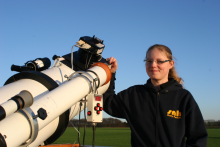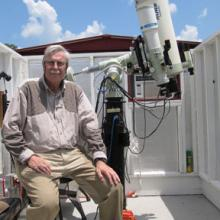# differential vs. absolute photometry

7 posts / 0 new
SNH01differential vs. absolute photometry

Hi,

I just started working myself through the Book "A Practical Guide to Lightcurve Photometry and Analysis" from Brian D. Warner.

There, he gives in many details, how to get absolute magnitudes from the desired objects. It is some more effort than the differential photometry described in the AAVSO CCD Manual.

Now my question: Why is the AAVSO method working so good? When I compare my (differential) measurements with those of others, they seem to be in very good agreement, although there have been no corrections for extinction, airmass and transform values.

Usually, it is said: if you want to compare measurements from different nights and different observers, you have to convert your differential magnitudes to the standard system. So you have to evaluate extinction, airmass and the transform values for your own system every night.

So when is it REALLY worth the effort for absolute photometry? Only when I want to study astroid lightcurves?

greetings
Katy

HOAWhy is the AAVSO method working so well?

Ignore

HOAWhy is the AAVSO method working so well?

Hi Katy,

Let me see if I can shed some light. It's helpful to work through these things.

Definitions

Upper case (capital) letter is what a "standard observer" measures. (V)

Lower case (small) letter is what you measure with your equipment. (v)

Subscript * is the variable star.  Subscript c is the comparison star.

k is the first order extinction coefficient.

X is the air mass.

T is the transformation coefficient.

Transformation Equation

Transformation from instrumental magnitude (v) to standard magnitude (V) is a function of air mass (X) and color (B-V). The transformation equation is V = v - kX + T(B-V) + constant. Now, the transformation equations for the variable star (*) and the comparison star (c) look like this:

V* = v* - kX* + T(B-V)* + constant

Vc = vc - kXc + T(B-V)c + constant

Rearrange the equations a little bit to put your observed magnitudes on the left side:

v* = V* + kX* - T(B-V)* - constant

vc = Vc + kXc - T(B-V)c  - constant

Now, calculate the difference between your observations of the variable star (*) and the comparison star (c):

v* - vc = (V* - Vc) + k(X* - Xc) - T [(B-V)* - (B-V)c] - [constant - constant]

Clearly, the constant term cancels out. (Simplification #1)

Extinction Term Cancels Out (Simplification #2)

In differential photometry, the variable star (*) and comparison star (c) are very close together in the sky. This means their air masses are practically the same. The result is that X* - Xc = 0 (almost exactly). So, the extinction term, k(X* - Xc),  cancels out!

The difference of instrumental magnitudes reduces to this:

v* - vc = (V* - Vc) - T[(B-V)* - (B-V)c]

Color Term Cancels Out (Simplification #3)

If the variable star (*) and comparison star (c) are chosen to have the same color, then (B-V)* = (B-V)c, and the color term also cancels out!

Why Everyone Gets Nearly the Same Result

After canceling the extinction and color terms, the result is v* - vc = V* - Vc. The magnitude difference you measure is identical to what a standard observer measures!

If this is true for one observer, then it may be true for most observers. This explains why different observers can get nearly the same results, even without transformation.

Complication - What if Colors Aren't the Same?

If the variable star (*) and comparison star (c) don't have the same color, then things aren't quite so simple. The practical worst case is that the color difference (B-V)* - (B-V)c = 2.0 magnitudes. Fortunately, the transformation coefficient is typically rather small, say T= 0.1. This is because the spectral response of your equipment probably isn't horribly different from the standard system. You do use an astronomical V filter and an astronomical CCD camera, don't you? Therefore, the color term might equal (0.1)(2.0) = 0.2 magnitude in the "practical worst" case. Unless you transform your observations, this is the bias that your data would have compared to what a standard observer measures.

If another observer uses the same equipment as you (Example - you both have a V filter from the same supplier, and you both use the same type CCD camera), then you might still get the same results, even if they are biased. Again, different observers may agree even if they don't transform the data.

Is it worth it to transform your observations? Without transformation, you can get very consistent results, though they may have bias. For example, you may get results that are systematically 0.1 magnitude fainter than the standard system. Another way to see this bias is if your measurements are systematically above or below the AAVSO light curve.

If you just want to achieve comparability with visual observers, it may not be necessary to transform. If you want to determine the minimum of an eclipsing binary, transformation probably isn't necessary. But if an asteroid and comparison star are separated by several degrees or more, and you are observing over a wide range of air masses, then you will want to transform.

Using comparison stars close to the variable and similar in color are reasons why differential photometry ("the AAVSO method") can work so well.

Hope this helps to clarify and that my equations are correct.

Clear skies, and good observing!

- Andy

SNH01Hi Andy, First of all:

Hi Andy,

First of all: Thank you very much for your detailed answer :-)

Yes, I do use a Johnson V-Filter (also B and R) and a SBIG ST-7. This ist probably the equipment, most of the other observers have.

Let's see, if I understood:
As long as I use the AAVSO-Comp stars, which are probably selected because they have similar colors as the variable, and my comp stars and variable is on the same image, the "AAVSO method" works fine.

What about cepheids? I read that they change color. Is that a lot? Does it make a big difference?

When I want to measure lightcurves of asteroids and I can find comp stars with a color index similar to our sun and they are on the same image as my asteroid, the "AAVSO method" should work here to?
Sometimes the magnitude range of an astroids light curve is very small. Should I then use rather the absolute photometry to get more accurate results?

Thank you
Katy

HOAComparison stars . . .

Hi Katy,

I don't think AAVSO comparison stars were selected because they have similar colors. They were chosen because they are close to the variable. The CCD photometry table for each chart includes stars with a wide range of colors. If the color of a comparison star differs significantly from the variable, then there will be a bias in the computed V magnitude. For highest accuracy, it would be necessary to do the complete transformation that accounts for the color difference between the comparison star and the variable star.

The AAVSO method of differential photometry can be as accurate as one wants to make it. The simplest way is to just observe through the V filter and not worry about transformations. This will give results that are accurate to within 0.1 - 0.2 magnitude of what a "standard observer" would measure. For highest accuracy, one would want to do the color transformations. I think this is what you mean by "absolute photometry." One should observe in two or more colors and then do the transformations. VPHOT makes it possible to do this.

I don't know about the color variation of Cepheid variables. If this is a concern, you could use the B, V, and R filters to measure the instrumental colors and see if they vary as a function of phase. For highest accuracy, take these measurements and then do the transformations.

If magnitude variation of an asteroid is small, then differential photometry would give better results. The advantage of differential photometry (the "AAVSO method") is that the comparison star is in the same field of view. Even if there are light clouds passing through the field, you will have better results than trying to use a comparison star somewhere else in the sky. For the most accurate results, observe in multiple colors and do the transformations.

You don't have to choose between the "AAVSO method" and the "absolute photometry" method. Both are compatible with each other. You can use the AAVSO method of differential photometry and then apply the transformations to get the most accurate results. Assuming you know the transformation coefficients,  VPHOT will do all the work. The result will be the standard ("absolute") magnitude(s) of what you are observing.

- Andy

SNH01waiting for clear skies

Hi Andy,

This sounds good.

I just have to wait for clear skies (which can be very long here in germany ) and then make some test-measurements to compare both methods. I will come back here, if I have more questions then

clear skies
katy

WBYTerminology Clarification

One does not obtain absolute magnitudes from absolute photometry. The term absolute magnitude has one meaning: The luminosity (or luminosity in a specific passband when specified) that one would measure at a distance of 10 parsecs. So if you refer to absolute V magnitude of a star it is the magnitude in the V band measured at a distance of 10 PC from the star. A capital M is also frequently used to denote absolute magnitude. So although V (capitalized) refers to a v magnitude properly transformed onto the standard Johnson Cousins system, Mv typically means the absolute magnitude in the V band.